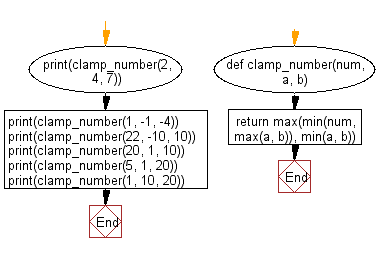﻿ Python Math: Clamps number within the inclusive range - w3resource# Python Math: Clamps number within the inclusive range

## Python Math: Exercise-87 with Solution

Write a Python program to cap a number within the inclusive range specified by the given boundary values x and y.

• If num falls within the range (a, b), return num.
• Otherwise, return the nearest number in the range.

Sample Solution:

Python Code:

``````def clamp_number(num, a, b):
return max(min(num, max(a, b)), min(a, b))

print(clamp_number(2, 4, 7))
print(clamp_number(1, -1, -4))
print(clamp_number(22, -10, 10))
print(clamp_number(20, 1, 10))
print(clamp_number(5, 1, 20))
print(clamp_number(1, 10, 20))
```
```

Sample Output:

```4
-1
10
10
5
10
```

Flowchart:## Visualize Python code execution:

The following tool visualize what the computer is doing step-by-step as it executes the said program:

Python Code Editor:

Have another way to solve this solution? Contribute your code (and comments) through Disqus.

What is the difficulty level of this exercise?

Test your Programming skills with w3resource's quiz.

﻿

```>>> students = [{'name': 'John', 'score': 98}, {'name': 'Mike', 'score': 94}, {'name': 'Jennifer', 'score': 99}]